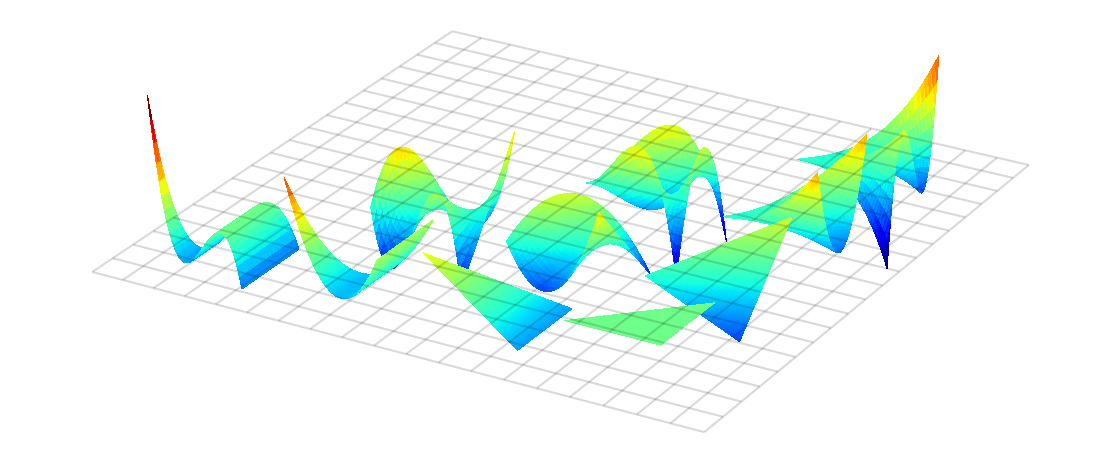# Welcome to modepy’s documentation!#

`modepy` helps you create well-behaved high-order discretizations on simplices (i.e. triangles and tetrahedra) and tensor products of simplices (i.e. squares, cubes, prisms, etc.). These are a key building block for high-order unstructured discretizations, as often used in a finite element context. It closely follows the approach taken in the book

Hesthaven, Jan S., and Tim Warburton. “Nodal Discontinuous Galerkin Methods: Algorithms, Analysis, and Applications”. 1st ed. Springer, 2007. Book web page

The basic objects that `modepy` manipulates are functions on a simplex. For example, it supplies an orthonormal basis on triangles (shown here) and tetrahedra.The file that created this plot is included in the `modepy` distribution as `examples/plot-basis.py`.

Here’s an idea of code that uses `modepy`:

```import numpy as np
import modepy as mp

# Define the shape and function space on which we will operate

n = 17  # use this total degree
dimensions = 2

shape = mp.Simplex(dimensions)
space = mp.PN(dimensions, n)

# Get a basis of orthonormal functions and some nodes.

basis = mp.orthonormal_basis_for_space(space, shape)
nodes = mp.edge_clustered_nodes_for_space(space, shape)
x, y = nodes

# We want to compute the x derivative of this function:

f = np.sin(5*x + y)
df_dx = 5 * np.cos(5*x + y)

# The (generalized) Vandermonde matrix transforms coefficients into
# nodal values. So we can find basis coefficients by applying its
# inverse:

vdm = mp.vandermonde(basis.functions, nodes)
f_coefficients = np.linalg.solve(vdm, f)

# Now linearly combine the (x-)derivatives of the basis using
# f_coefficients to compute the numerical derivatives.

This file is included in the `modepy` distribution as `examples/derivative.py`.